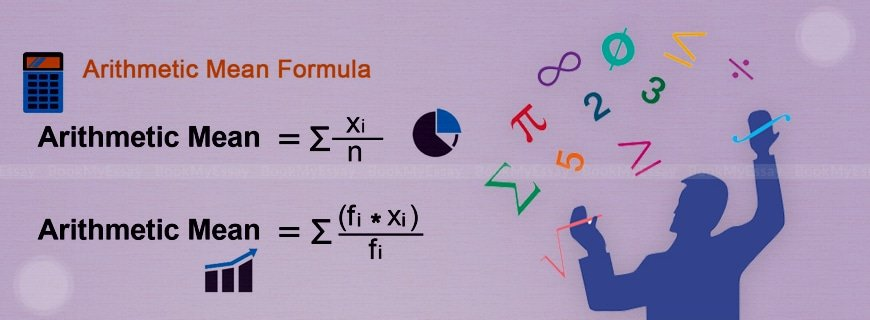# Arithmetic Assignment HelpGet a Free Quote
It's free and always will be.
Page - +
words
NWL

## Arithmetic Assignment Help

Arithmetic provides the basis of all modern mathematics. It is also the oldest mathematical form used in many real world activities. Since, it is the foundation of higher mathematics, students are taught arithmetic at the beginning before they get accustomed to other forms of mathematics like geometry and algebra. Students learning arithmetic are often given assignments and home tasks to solve in time and get assessed.

BookMyEssay provides all necessary Arithmetic assignment help to students who are struggling hard with their assignment or home tasks. Assignment help provided by BookMyEssay is considered as the most professional service in this industry that keeps thousands of students who contact mathematics assignment writing service frequently.

### Arithmetic: An overview

Students, especially at lower classes, are given lessons, to get accustomed with and apply different processes of arithmetic. This helps them to learn algebra at the next level more easily. Again, these arithmetical processes have wide array of applications in commerce and other branches of science. Arithmetic is a division of mathematics that deals with different properties of the whole numbers as well as fractions and the basic operations applicable to these numbers. This branch of mathematics mainly deals with addition, subtraction, multiplication and division of whole and fractional numbers. In arithmetic students also learn the use of brackets in factorization. Moreover, at higher levels of arithmetic, some important processes that are significant in different streams of studies and also important in day-to-day activities of normal human being are also taught in-depth. Some of these important arithmetical processes are as follows –

• Percentage – In mathematics percentage is a ratio taking 100 as the base. That simply means, it is fraction whose denominator is always 100. The number that comes in numerator is expressed with the symbol ‘%’. If it is asked, 5 students in a class of 50 scored more than 90, then what percentage of students scored more than 90, then the students need to use unitary method to find how many students scored more than 90 if there were 100 students. Thus, percentage is worked out. Here the answer is 10%.
• Unitary method – It is a very useful and universally accepted method of finding a value by at first finding the value of a single unit. Like in the above example if out of 50 students only 5 scored more than 90, then it is to be calculated how many students score more than 90 if there are only on student in the class, then how many students scored more than 90 if there were 100 students. The process applied is called unitary method.
• Progression – There are basically two types of progressions found in number series. Firstly, arithmetic progression where any two consecutive numbers in a series have a common factor which is to be added with the previous number to get the next number. Secondly, geometric progression where two consecutive numbers in a series have a fixed quotient.

Besides, students are also given lessons on number line, properties of numbers, properties of fractions, ratio and proportions, square roots, time and distance, recurring decimals, reduction and expansion method, and properties of integers etc.

### Importance of arithmetic lessons and assignments

As it is said earlier, arithmetic is the foundation of higher mathematics as also foundation of many practical calculations that are required in day-to-day activities. But, it is a fact that two few students actually show any interest in getting in-depth knowledge on arithmetic. However, they are bound to learn the subject and bound to face assignments in different forms. Teachers provide different types of assignments to test how their students are learning different arithmetical methods and how efficiently they are applying these methods in different problems.

### Mental stress associated with arithmetic assignments

Most of the students feel highly nervous while completing arithmetic assignment of their own. Arithmetic assignments mostly involve complex calculations with the help of more than one methods, like introduction of arithmetic progression and square root in a single assignment or factorization of fractions etc. Due to lack of understanding on different mathematical processes and lack of interest on the subject, students feel too nervous to solve these problems of their own. There nervousness multiplies when deadlines are too short. In such circumstances, arithmetic assignment help appears very supportive and necessary.

### Types of assignments

At higher classes assignments are too serious affairs. Those are given with some guidelines and specific deadlines, while students at lower classes are given mathematical assignment writing in form of worksheets.

### Help extended by BookMyEssay

BookMyEssay extends all necessary help with to students getting hopeless with their arithmetic assignments –

• The organization provides most experienced arithmetic experts who have in-depth knowledge on all methods and theories of arithmetic.